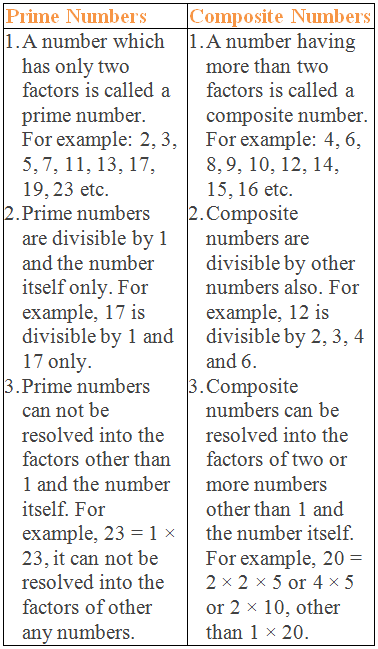# Prime or Composite?### What are Prime and Composite Numbers?

A number which has only two factors is called a Prime Number and a number having more than two factors is called a Composite Number.

********************

10 Math Problems officially announces the release of Quick Math Solver, an Android App on the Google Play Store for students around the world.

********************For example,

2 has only two factors 1 and 2, so 2 is a prime number.

3 has only two factors 1 and 3, so 3 is a prime number.

5 has only two factors 1 and 5, so 5 is a prime number.

etc. But,

4 has three factors 1, 2, and 4, so 4 is a composite number.

6 has four factors 1, 2, 3, and 6, so 6 is a composite number.

8 has four factors 1, 2, 4, and 8, so 8 is a composite number.

etc.

### What are the differences between prime vs composite numbers?

Prime VS Composite Numbers### Is 0 a prime or composite number?

0 is neither a prime nor a composite number.

### Is 1 a prime or composite number?

1 is neither a prime nor a composite number.

Is 2 prime or composite number?

2 is a prime number.

### Is 3 a prime or composite number?

3 is a prime number.

### Is 4 a prime or composite number?

4 is a composite number.

### Is 5 a prime or composite number?

5 is a prime number.

### Is 6 a prime or composite number?

6 is a composite number.

### Is 7 a prime or composite number?

7 is a prime number.

### Is 8 a prime or composite number?

8 is a composite number.

Is 9 prime or composite?

9 is a composite number.

### Is 12 prime or composite?

12 is a composite number.

### Is 13 prime or composite?

13 is a prime number.

### Is 15 prime or composite?

15 is a composite number.

### Is 17 prime or composite?

17 is a prime number.

### Is 19 prime or composite?

19 is a prime number.

### Is 21 prime or composite?

21 is a composite number.

### Is 23 prime or composite?

23 is a prime number.

### Is 29 prime or composite?

29 is a prime number.

### Is 31 prime or composite?

31 is a prime number.

### Is 49 a prime or composite number?

49 is a composite number.

### Is 51 prime or composite?

51 is a composite number.

### Is 91 a prime or composite number?

91 is a composite number.

### Which numbers are neither prime nor composite?

0 and 1 are neither prime nor composite numbers.